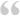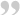hmmm, I'm not getting a positive result with that either.

php:
``````<?php
\$min =5;
\$max =12;
if ((\$min %2 = 0 AND \$max %2 = 0) OR (\$min %2 != 0 AND \$max %2 != 0)) {
} elseif ((\$min %2 = 0 AND \$max %2 != 0) OR (\$min %2 !=0 AND \$max %2 = 0)) {
\$max = \$max+1;
}
echo "\$min";
echo "\$max";
?>``````

The above code produces the following error...Parse error: syntax error, unexpected '=' on line 3The following code will produce an echo result of "3 is odd"

php:
``````<?php
\$i=3;
if (\$i % 2) {
echo "\$i is odd";
} else {
echo "\$i is even";
}
?>``````

So I'm still baffled on syntax...